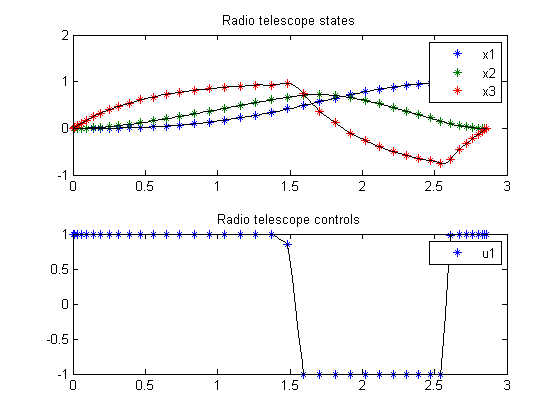90.1  Problem description

Time-optimal positioning of a radio telescope with bounded control.

Programmers: Gerard Van Willigenburg (Wageningen University) Willem De Koning (retired from Delft University of Technology)

90.2  Problem setup

% Array with consecutive number of collocation points
narr = [20 40];

% Free final time
toms t t_f

for n=narr
p = tomPhase('p', t, 0, t_f, n);
setPhase(p)

tomStates x1 x2 x3
tomControls u1

% Initial & terminal states
xi = [0; 0; 0];
xf = [1; 0; 0];

% Initial guess
if n==narr(1)
x0 = {t_f == 5; icollocate({x1 == xi(1); x2 == xi(2)
x3 == xi(3)})
collocate({u1 == 0})};
else
x0 = {t_f == tfopt; icollocate({x1 == xopt1; x2 == xopt2
x3 == xopt3})
collocate({u1 == uopt1})};
end

% Box constraints
cbox = {-1 <= collocate(u1) <= 1, 0.1 <= t_f <= 10};

% Boundary constraints
cbnd = {initial({x1 == xi(1); x2 == xi(2); x3 == xi(3)})
final({x1 == xf(1); x2 == xf(2); x3 == xf(3)})};

% ODEs and path constraints
dx1 = x2;
dx2 = -0.5*x2-0.1*x2./sqrt(x2.*x2+1e-4)+x3;
dx3 = -2*x3+2*u1;

ceq = collocate({
dot(x1) == dx1
dot(x2) == dx2
dot(x3) == dx3});

% Objective
objective = t_f;

90.3  Solve the problem

options = struct;
solution = ezsolve(objective, {cbox, cbnd, ceq}, x0, options);

tfopt = subs(t_f,solution);
xopt1 = subs(x1,solution);
xopt2 = subs(x2,solution);
xopt3 = subs(x3,solution);
uopt1 = subs(u1,solution);
Problem type appears to be: lpcon
Starting numeric solver
===== * * * =================================================================== * * *
TOMLAB - Tomlab Optimization Inc. Development license  999001. Valid to 2011-02-05
=====================================================================================
Problem: ---  1: Radio telescope                f_k       2.866900592275516900
sum(|constr|)      0.000001022940510087
f(x_k) + sum(|constr|)      2.866901615216026900
f(x_0)      5.000000000000000000

Solver: snopt.  EXIT=0.  INFORM=1.
SNOPT 7.2-5 NLP code
Optimality conditions satisfied

FuncEv    1 ConstrEv   41 ConJacEv   41 Iter   22 MinorIter  169
CPU time: 0.109375 sec. Elapsed time: 0.110000 sec.
Problem type appears to be: lpcon
Starting numeric solver
===== * * * =================================================================== * * *
TOMLAB - Tomlab Optimization Inc. Development license  999001. Valid to 2011-02-05
=====================================================================================
Problem: ---  1: Radio telescope                f_k       2.859991999288976800
sum(|constr|)      0.000002602280303807
f(x_k) + sum(|constr|)      2.859994601569280500
f(x_0)      2.866900592275516900

Solver: snopt.  EXIT=0.  INFORM=1.
SNOPT 7.2-5 NLP code
Optimality conditions satisfied

FuncEv    1 ConstrEv    5 ConJacEv    5 Iter    4 MinorIter  133
CPU time: 0.062500 sec. Elapsed time: 0.062000 sec.
end

figure(1)
subplot(2,1,1);
ezplot([x1; x2; x3]); legend('x1','x2','x3');